## 1.1 Smoothing

Smoothing is a descriptive statistical tool for summarizing data, a practical visualization technique, as well as a nonparametric estimation methodology. The basic idea is that data is representative of an underlying distribution with some smoothness properties, and we would like to approximate or estimate this underlying distribution from data.

There are two related but slightly different approaches. Either we attempt to estimate a smooth density of the observed variables, or we attempt to estimate a smooth conditional density of one variable given others. The latter can in principle be done by computing the conditional density from a smooth estimate of the joint density. Thus it appears that we really just need a way of computing smooth density estimates. In practice it may, however, be better to solve the conditional smoothing problem directly instead of solving a strictly more complicated problem. This is particularly so, if the conditioning variables are fixed e.g. by a design, or if our main interest is in the conditional mean or median, say, and not the entire conditional distribution. Conditional smoothing is dealt with in Chapter 3.

In this introduction we focus on the univariate case, where there really only is one problem: smooth density estimation. Moreover, this is a very basic problem, and one viewpoint is that we simply need to “smooth out the jumps of the histogram”. Indeed, it does not need to be made more sophisticated than that! Humans are able to do this quite well using just a pen and a printed histogram, but it is a bit more complicated to automatize such a smoothing procedure. Moreover, an automatized procedure is likely to need calibration to yield a good tradeoff between smoothness and data fit. This is again something that humans can do quite well by eyeballing visualizations, but that approach does not scale, neither in terms of the number of density estimates we want to consider, nor in terms of going from univariate to multivariate densities.

If we want to really discuss how a smoothing procedure works not just as a heuristic but also as an estimator of an underlying density, it is necessary to formalize how to quantify the performance of the procedure. This increases the level of mathematical sophistication, but it allows us to discuss optimality, and it lets us develop fully automatized procedures that do not rely on human calibration. While human inspection of visualizations is always a good idea, computational statistics is also about offloading humans from all computational tasks that can be automatized. This is true for smoothing as well, hence the need for automatic and robust smoothing procedures that produce well calibrated results with a minimum of human effort.

### 1.1.1 Angle distributions in proteins

We will illustrate smoothing using a small data set on angles formed between two subsequent peptide planes in 3D protein structures. This data set is selected because the angle distributions are multimodal and slightly non-standard, and these properties are well suited for illustrating fundamental considerations regarding smooth density estimation in practice.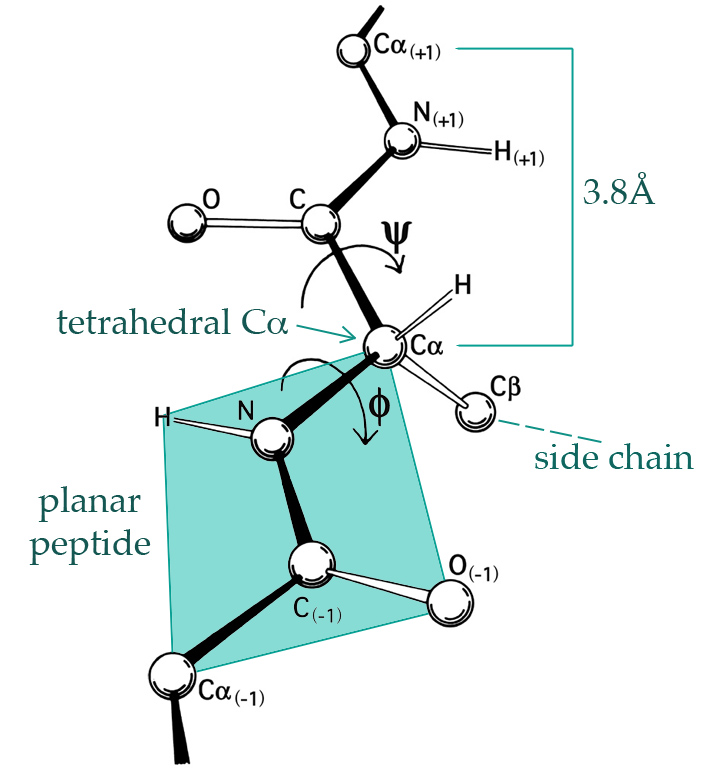Figure 1.1: The 3D structure of proteins is largely given by the $$\phi$$- and $$\psi$$-angles of the peptide planes. (By Dcrjsr, CC BY 3.0 via Wikimedia Commons.)

A protein is a large molecule consisting of a backbone with carbon and nitrogen atoms arranged sequentially: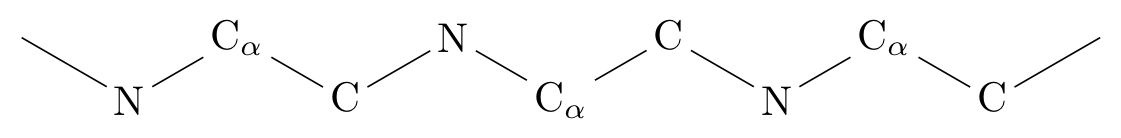A hydrogen atom binds to each nitrogen (N) and an oxygen atom binds to each carbon without the $$\alpha$$ subscript (C), see Figure 1.1, and such four atoms form together what is known as a peptide bond between two alpha-carbon atoms (C$$_{\alpha}$$). Each C$$_{\alpha}$$ atom binds a hydrogen atom and an amino acid side chain. There are 20 naturally occurring amino acids in genetically encoded proteins, each having a three letter code (such as Gly for Glycine, Pro for Proline, etc.). The protein will typically form a complicated 3D structure determined by the amino acids, which in turn determine the $$\phi$$- and the $$\psi$$-angles between the peptide planes as shown on Figure 1.1.

We will consider a small data set, phipsi, of experimentally determined angles from a single protein, the human protein 1HMP, which is composed of two chains (denoted A and B). Figure 1.2 shows the 3D structure of the protein.

head(phipsi)
##   chain  AA pos        phi        psi
## 1     A Pro   5 -1.6218794  0.2258685
## 2     A Gly   6  1.1483709 -2.8314426
## 3     A Val   7 -1.4160220  2.1190570
## 4     A Val   8 -1.4926720  2.3941331
## 5     A Ile   9 -2.1814653  1.4877618
## 6     A Ser  10 -0.7525375  2.5676186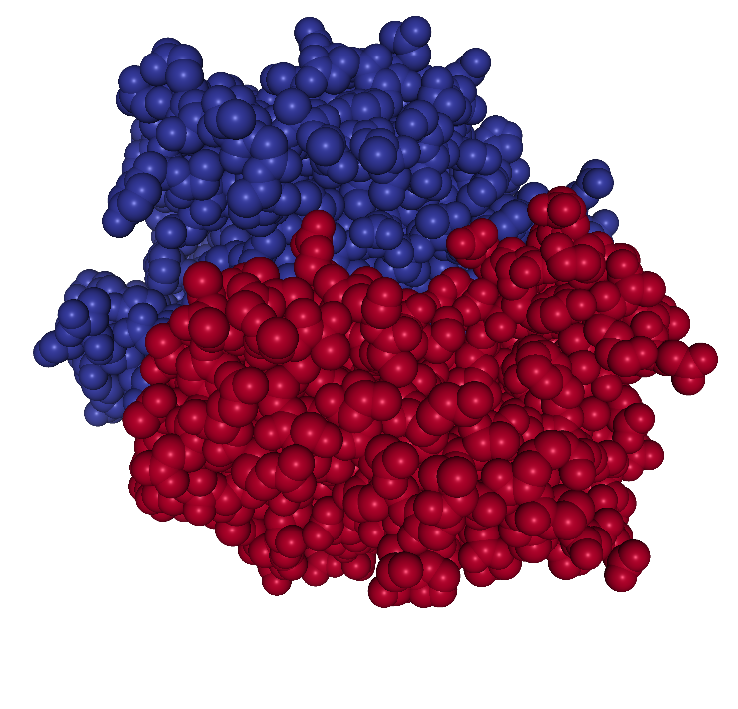Figure 1.2: The 3D structure of the atoms constituting the protein 1HMP. The colors indicate the two different chains.

We can use base R functions such as hist and density to visualize the marginal distributions of the two angles.

hist(phipsi$phi, prob = TRUE) rug(phipsi$phi)
density(phipsi$phi) %>% lines(col = "red", lwd = 2) hist(phipsi$psi, prob = TRUE)
rug(phipsi$psi) density(phipsi$psi) %>% lines(col = "red", lwd = 2)Figure 1.3: Histograms equipped with a rug plot and smoothed density estimate (red line) of the distribution of $$\phi$$-angles (left) and $$\psi$$-angles (right).

The smooth red density curve shown in Figure 1.3 can be thought of as a smooth version of a histogram. It is surprisingly difficult to find automatic smoothing procedures that perform uniformly well – it is even quite difficult to automatically select the number and positions of the breaks used for histograms. This is one of the important points that is taken up in this book: how to implement good default choices of various tuning parameters that are required by any smoothing procedure.

### 1.1.2 Using ggplot2

It is also possible to use ggplot2 to achieve similar results.

library(ggplot2)
ggplot(phipsi, aes(x = phi)) +
geom_histogram(aes(y = ..density..), bins = 13) +
geom_density(col = "red", size = 1) +
geom_rug()

ggplot(phipsi, aes(x = psi)) +
geom_histogram(aes(y = ..density..), bins = 13) +
geom_density(col = "red", size = 1) +
geom_rug()Figure 1.4: Histograms and density estimates of $$\phi$$-angles (left) and $$\psi$$-angles (right) made with ggplot2.

Histograms produced by ggplot2 have a non adaptive default number of bins equal to 30 (number of breaks equal to 31), which is different from hist that uses Sturges’ formula $\text{number of breaks} = \lceil \log_2(n) + 1 \rceil$ with $$n$$ the number of observations in the data set. In addition, this number is further modified by the function pretty that generates “nice” breaks, which results in 14 breaks for the angle data. For easier comparison, the number of bins used by geom_histogram above is set to 13, though it should be noticed that the breaks are not chosen in exactly the same way by geom_histogram and hist. Automatic and data adaptive bin selection is difficult, and geom_histogram implements a simple and fixed, but likely suboptimal, default while notifying the user that this default choice can be improved by setting binwidth.

For the density, geom_density actually relies on the density function and its default choices of how and how much to smooth. Thus the figure may have a slightly different appearance, but the estimated density obtained by geom_density is identical to the one obtained by density.

### 1.1.3 Changing the defaults

The range of the angle data is known to be $$(-\pi, \pi]$$, which neither the histogram nor the density smoother take advantage of. The pretty function, used by hist chooses, for instance, breaks in $$-3$$ and $$3$$, which results in the two extreme bars in the histogram to be misleading. Note also that for the $$\psi$$-angle it appears that the defaults result in oversmoothing of the density estimate. That is, the density is more smoothed out than the data (and the histogram) appears to support.

To obtain different – and perhaps better – results, we can try to change some of the defaults of the histogram and density functions. The two most important defaults to consider are the bandwidth and the kernel. Postponing the mathematics to Chapter 2, the kernel controls how neighboring data points are weighted relatively to each other, and the bandwidth controls the size of neighborhoods. A bandwidth can be specified manually as a specific numerical value, but for a fully automatic procedure, it is selected by a bandwidth selection algorithm. The density default is a rather simplistic algorithm known as Silverman’s rule-of-thumb.

hist(phipsi$psi, breaks = seq(-pi, pi, length.out = 15), prob = TRUE) rug(phipsi$psi)
density(phipsi$psi, adjust = 1, cut = 0) %>% lines(col = "red", lwd = 2) density(phipsi$psi, adjust = 0.5, cut = 0) %>% lines(col = "blue", lwd = 2)
density(phipsi$psi, adjust = 2, cut = 0) %>% lines(col = "purple", lwd = 2) hist(phipsi$psi, breaks = seq(-pi, pi, length.out = 15), prob = TRUE)
rug(phipsi$psi) # Default kernel is "gaussian" density(phipsi$psi, bw = "SJ", cut = 0) %>% lines(col = "red", lwd = 2)
density(phipsi$psi, kernel = "epanechnikov", bw = "SJ", cut = 0) %>% lines(col = "blue", lwd = 2) density(phipsi$psi, kernel = "rectangular", bw = "SJ", cut = 0) %>%
lines(col = "purple", lwd = 2)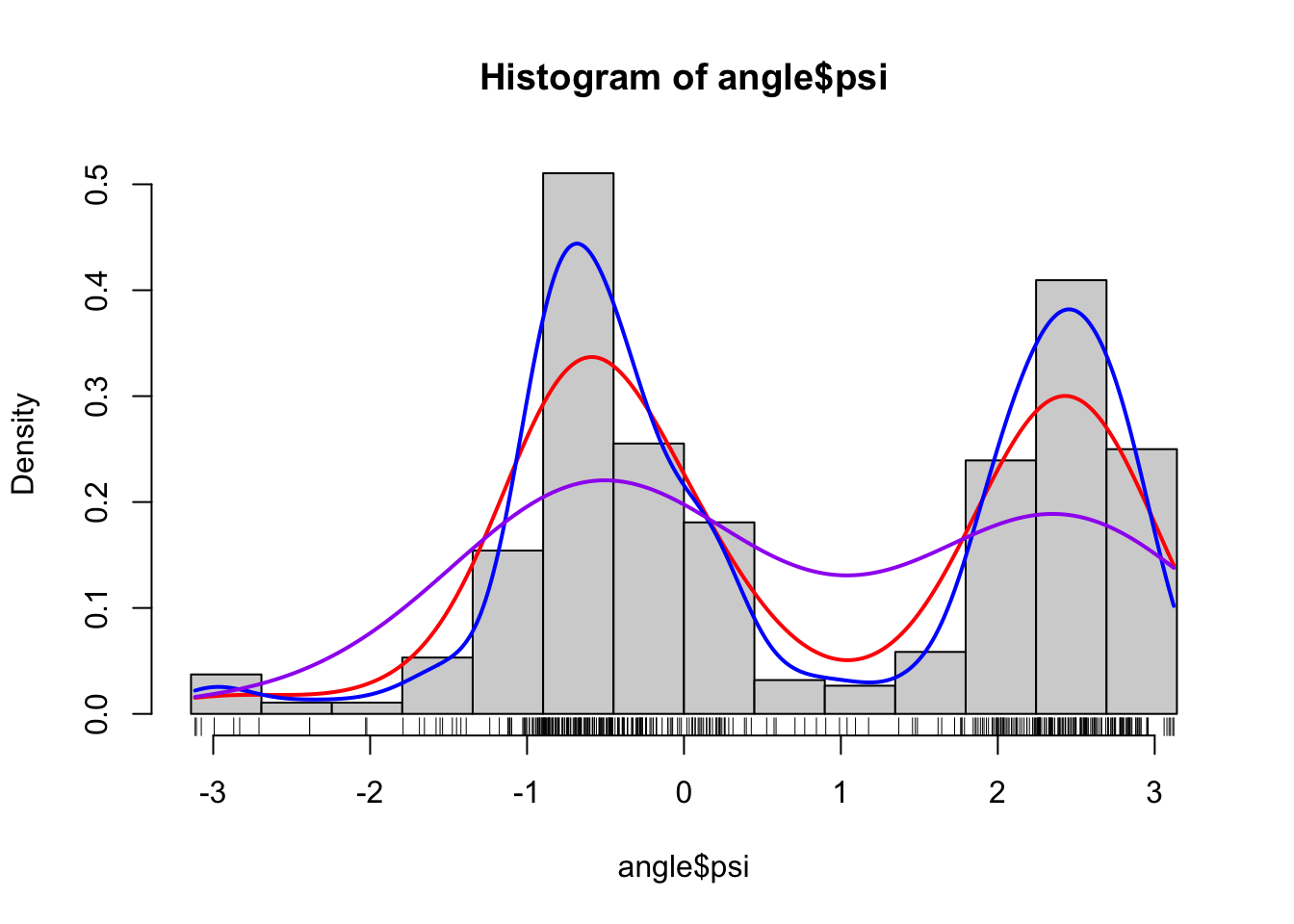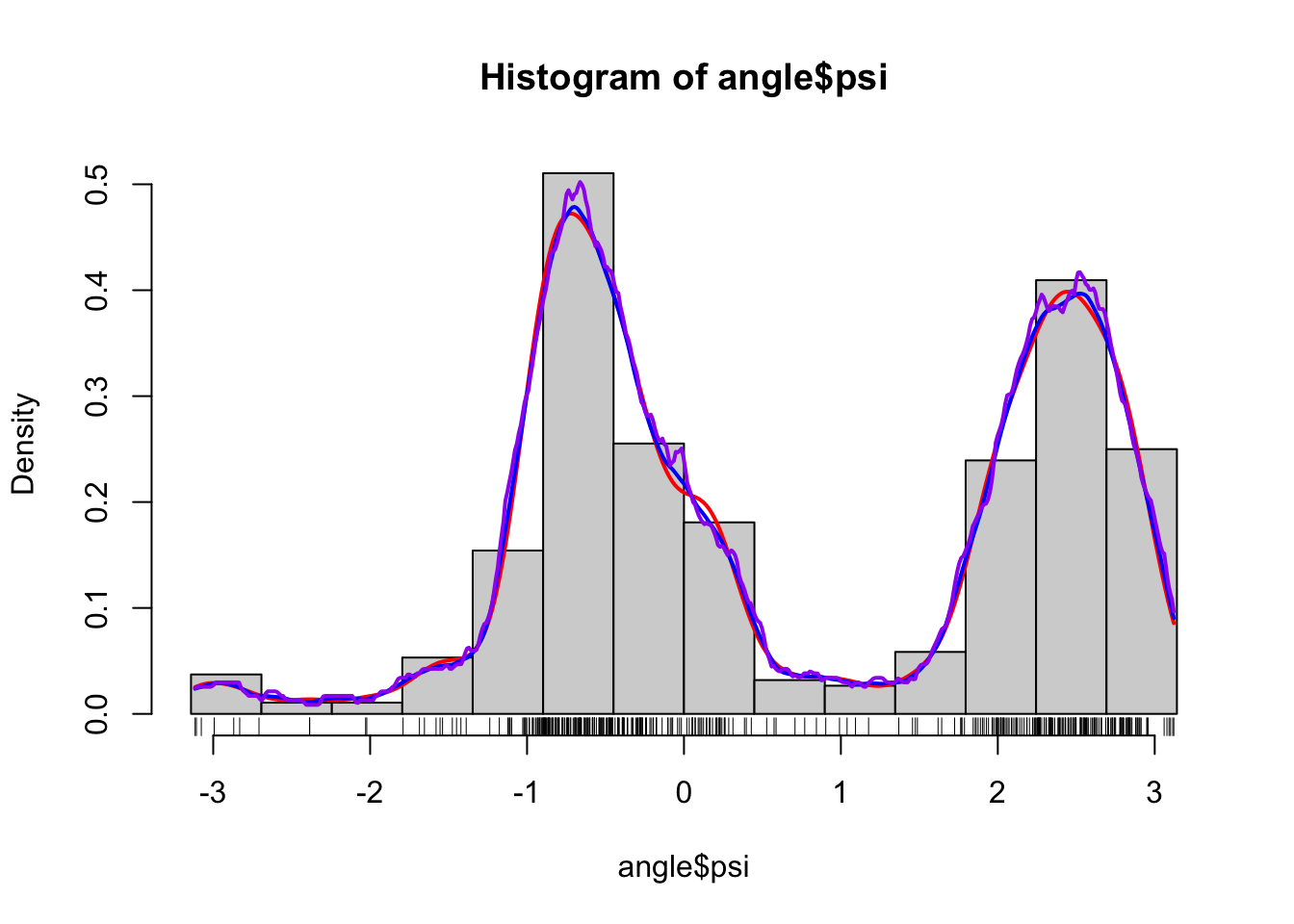Figure 1.5: Histograms and various density estimates for the $$\psi$$-angles. The colors indicate different choices of bandwidth adjustments using the otherwise default bandwidth selection (left) and different choices of kernels using Sheather-Jones bandwidth selection (right).

Figure 1.5 shows examples of several different density estimates that can be obtained by changing the defaults of density. The breaks for the histogram have also been chosen manually to make sure that they match the range of the data. Note, in particular, that Sheather-Jones bandwidth selection appears to work better than the default bandwidth for this example. This is generally the case for multimodal distributions, where the default bandwidth tends to oversmooth. Note also that the choice of bandwidth is far more consequential than the choice of kernel, the latter mostly affecting how wiggly the density estimate is locally.

It should be noted that defaults arise as a combination of historically sensible choices and backward compatibility. Thought should go into choosing a good, robust default, but once a default is chosen, it should not be changed haphazardly, as this might break existing code. That is why not all defaults used in R are by today’s standards the best known choices. You see this argument made in the documentation of density regarding the default for bandwidth selection, where Sheather-Jones is suggested as a better default than the current, but for compatibility reasons Silverman’s rule-of-thumb is the default and is likely to remain being so.

### 1.1.4 Multivariate methods

This section provides a single illustration of how to use the bivariate kernel smoother kde2d from the MASS package for bivariate density estimation of the $$(\phi, \psi)$$-angle distribution. A scatter plot of $$\phi$$ and $$\psi$$ angles is known as a Ramachandran plot, and it provides a classical and important way of visualizing local structural constraints of proteins in structural biochemistry. The density estimate can be understood as an estimate of the distribution of $$(\phi, \psi)$$-angles in naturally occuring proteins from the small sample of angles in our data set.

We compute the density estimate in a grid of size 100 by 100 using a bandwidth of 2 and using the kde2d function that uses a bivariate normal kernel.

denshat <- MASS::kde2d(phipsi$phi, phipsi$psi, h = 2, n = 100)
denshat <- data.frame(
cbind(
denshat$x, rep(denshat$y, each = length(denshat$x)), as.vector(denshat$z)
)
)
colnames(denshat) <- c("phi", "psi", "dens")
p <- ggplot(denshat, aes(phi, psi)) +
geom_tile(aes(fill = dens), alpha = 0.5) +
geom_contour(aes(z = sqrt(dens))) +
geom_point(data = phipsi, aes(fill = NULL)) +
scale_fill_gradient(low = "white", high = "darkblue", trans = "sqrt")

We then recompute the density estimate in the same grid of size using the smaller bandwidth of 0.5.

denshat <- MASS::kde2d(phipsi$phi, phipsi$psi, h = 0.5, n = 100)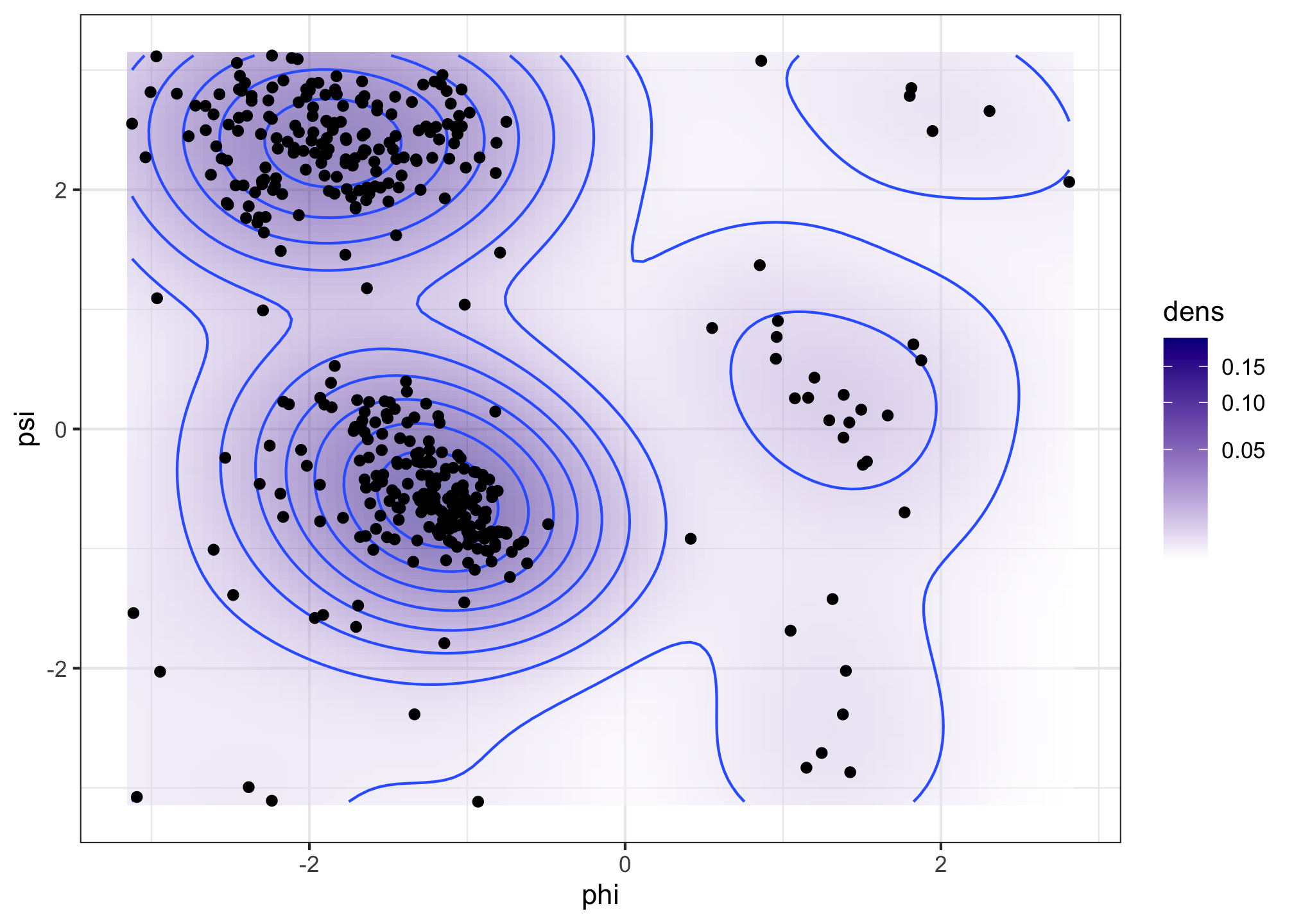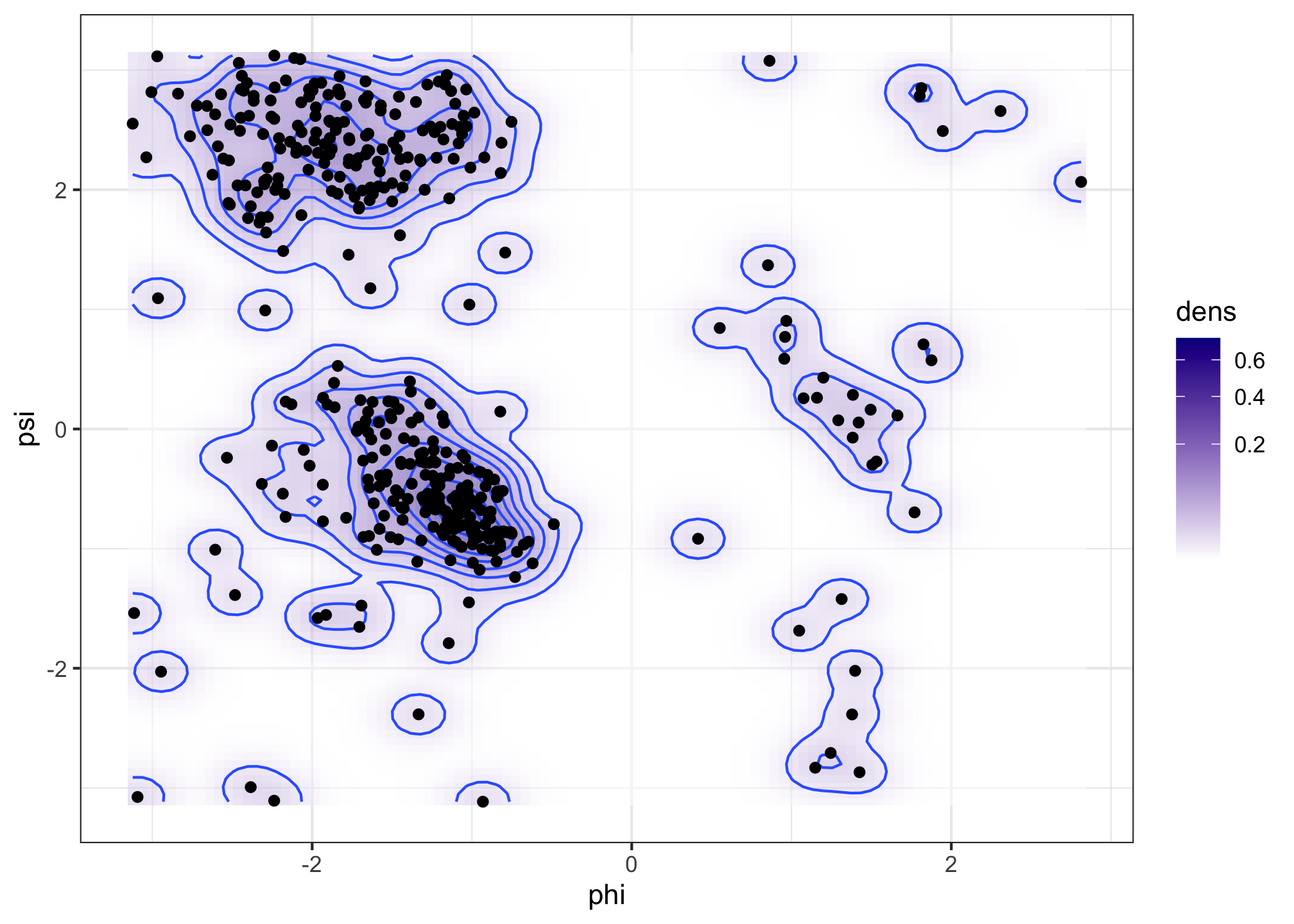Figure 1.6: Bivariate density estimates of protein backbone angles using a bivariate Gaussian kernel with bandwiths $$2$$ (left) and $$0.5$$ (right).

The Ramachandran plot in Figure 1.6 shows how structural constraints of a protein, such as steric effects, induce a non-standard bivariate distribution of $$(\phi, \psi)$$-angles.

### 1.1.5 Large scale smoothing

With small data sets of less than 10,000 data points, say, univariate smooth density estimation requires a very modest amount of computation. That is true even with rather naive implementations of the standard methods. The R function density is implemented using a number of computational tricks like binning and the fast Fourier transform, and it can compute density estimates with a million data points (around 8 MB) within a fraction of a second.

It is unclear if we ever need truly large scale univariate density estimation with terabytes of data points, say. If we have that amount of (heterogeneous) data it is likely that we are better off breaking the data down into smaller and more homogeneous groups. That is, we should turn a big data computation into a large number of small data computations. That does not remove the computational challenge but it does diminish it somewhat e.g. by parallelization.

Deng and Wickham did a review in 2011 on Density estimation in R, where they assessed the performance of a number of R packages including the density function. The KernSmooth package was singled out in terms of speed as well as accuracy for computing smooth density estimates with density performing quite well too. (Histograms are non-smooth density estimates and generally faster to compute). The assessment was based on using defaults for the different packages, which is meaningful in the sense of representing the performance that the occasional user will experience. It is, however, also an evaluation of the combination of default choices and the implementation, and as different packages rely on e.g. different bandwidth selection algorithms, this assessment is not the complete story. The bkde function from the KernSmooth package, as well as density, are solid choices, but the point is that performance assessment is a multifaceted problem.

To be a little more specific about the computational complexity of density estimators, suppose that we have $$n$$ data points and want to evaluate the density in $$m$$ points. A naive implementation of kernel smoothing, Section 2.2, has $$O(mn)$$ time complexity, while a naive implementation of the best bandwidth selection algorithms have $$O(n^2)$$ time complexity. As a simple rule-of-thumb, anything beyond $$O(n)$$ will not scale to very large data sets. A quadratic time complexity for bandwidth selection will, in particular, be a serious bottleneck. Kernel smoothing illustrates perfectly that a literal implementation of the mathematics behind a statistical method may not always be computationally viable. Even the $$O(mn)$$ time complexity may be quite a bottleneck as it reflects $$mn$$ kernel evaluations, each being potentially a computationally relatively expensive operation.

The binning trick, with the number of bins set to $$m$$, is a grouping of the data points into $$m$$ sets of neighbor points (bins) with each bin representing the points in the bin via a single point and a weight. If $$m \ll n$$, this can reduce the time complexity substantially to $$O(m^2) + O(n)$$. The fast Fourier transform may reduce the $$O(m^2)$$ term even further to $$O(m\log(m))$$. Some approximations are involved, and it is of importance to evaluate the tradeoff between time and memory complexity on one side and accuracy on the other side.

Multivariate smoothing is a different story. While it is possible to generalize the basic ideas of univariate density estimation to arbitrary dimensions, the curse-of-dimensionality hits unconstrained smoothing hard – statistically as well as computationally. Multivariate smoothing is therefore still an active research area developing computationally tractable and novel ways of fitting smooth densities or conditional densities to multivariate or even high-dimensional data. A key technique is to make structural assumptions to alleviate the challenge of a large dimension, but there are many different assumptions possible, which makes the body of methods and theory richer and the practical choices much more difficult.